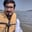a concise shot of dev knowledge
Become a Contributor
Concise shots of dev knowledge

RELATED TAGS

communitycreator
machine learning

# What is the tradeoff between Bias and Variance?Arslan Bajwa

## Bias

The difference between the average value predicted by our Machine Learning model and the correct target value is known as Bias. A model that makes incorrect predictions about a dataset is called a biased model. This model oversimplifies the target function to make it easier to learn.

High Bias / Underfitting

### Characteristics of a High Bias Model

1. Underfitting: A model with High Bias tends to underfit the data as it oversimplifies the solution by failing to learn how to train the data efficiently. This results in a linear function.

2. Oversimplification: Due to the model being too simple, the biased model is unable to learn complex features of a training data, thus, making it inefficient when solving complex problems.

3. Low Training Accuracy: Due to the inability to correctly process training data, the biased model shows high-training loss resulting in low-training accuracy.

### Solution to High Bias

High bias happens because of a high training error. There are multiple ways to reduce the bias of a model, such as:

1. By adding more features from the data to make the model more complex.
2. By increasing training iterations so that more complex models and relevant data can be learned.
3. Replacing current model with more complex model can reduce the bias.
4. Using non-linear algorithms
5. Using non-parameterized algorithms
6. By decreasing regularization on inputs at different levels, the model can learn the training set more efficiently and prevent underfitting.
7. By using a new model architecture. However, this should only be used as a last resort if none of the methods above give satisfactory results.

## Variance

The amount of variability in the target function in response to a change in the training data is known as Variance. When a model takes into consideration the noise and fluctuation in the data, it is said to be of High Variance.

High Variance / Overfitting

### Characteristics of a High Variance Model

1. Overfitting: A model with High Variance tends to overfit the data as it overcomplicates the solution and fails to generalize new test data. This results in a non-linear function.

2. Overcomplication: Due to the model being too complex, the model learns a much more complex curve and fails to work efficiently on simple problems.

3. Low Testing Accuracy: Although these models tend to work well on training data with high accuracy, they fail to efficiently work on test data where they will show a huge test data loss.

### Solution to High Variance

High variance is due to a high validation error. There are multiple ways of reducing the variance of a model such as:

1. By reducing features from the data to make the model less complex
2. By increasing training data, complex models can be simplified in order to balance out the model complexity and amount of datasets.
3. Replacing current model with a simpler model can reduce the variance.
4. Using lower degree algorithms.
5. Using hyper parameter tuning to avoid overfitting
6. By increasing regularization on inputs at different levels, the model complexity will be reduced. This will prevent overfitting.
7. Use a new model architecture. However, this should only be used as a last resort if none of the methods above give satisfactory results.

As seen above, if the algorithm is too simple, it will have a high bias and a low variance. Similarly, if the algorithm is too complex, it will have a high variance and a low bias. Therefore, it is clear that:

“Bias and variance are complements of each other” The increase of one will result in the decrease of the other and vice versa. Hence, finding the right balance of values is known as the Bias-Variance Tradeoff.

Target Function

An ideal algorithm should neither underfit nor overfit the data. The end goal of all Machine Learning Algorithms is to produce a function that has both low-bias and low-variance.

Bias-Variance Graph

Hypothetically, the dotted line above is the required optimal solution. But, in the real world, it is very difficult to achieve due to an unknown best target function. The goal is to find an iterative process through which we can keep on improving our Machine Learning Algorithm so that its predictions will improve.

RELATED TAGS

communitycreator
machine learning

CREATORArslan Bajwa
RELATED COURSES

View all Courses

Keep Exploring

Learn in-demand tech skills in half the time